Learning Library
Who we are
Guidance

# 3.OA.C.7 Worksheets, Workbooks, Lesson Plans, and Games

#### CCSS.MATH.CONTENT.3.OA.C.7

:
"Fluently multiply and divide within 100, using strategies such as the relationship between multiplication and division (e.g., knowing that 8 × 5 = 40, one knows 40 ÷ 5 = 8) or properties of operations. By the end of Grade 3, know from memory all products of two one-digit numbers."

Our Take: By the end of third grade, students should have memorized their basic multiplication and division facts up to 100. Grasping these facts requires experiences that extend well beyond drills and timed tests. Students should have varied learning opportunities to discover patterns and relationships within multiplication facts and build a firm understanding of the relationship between multiplication and division. One fun and engaging related learning activity involves playing "Around the World" with multiplication fact flash cards.

These worksheets can help students practice this Common Core State Standards skill.

## Worksheets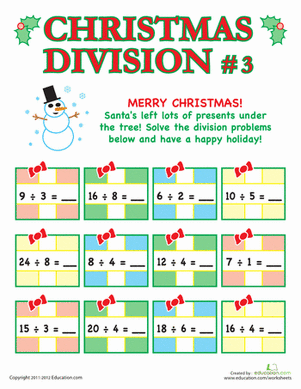Christmas Division #3
Worksheet
Christmas Division #3
Ho ho ho! Here are some colorful presents from Santa, filled with simple division problems for your third grader to solve.
Math
Worksheet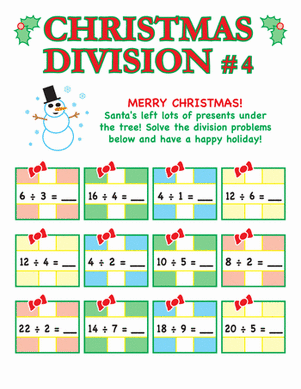Christmas Division #4
Worksheet
Christmas Division #4
Enjoy a festive math sheet for the Christmas season! This one has simple division presents in store to keep the learning going over the winter break.
Math
Worksheet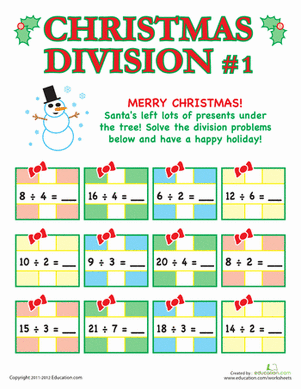Christmas Division #1
Worksheet
Christmas Division #1
Santa has brought a colorful page of simple division problems for your child to solve. Keep the learning going this holiday, but still fun!
Math
Worksheet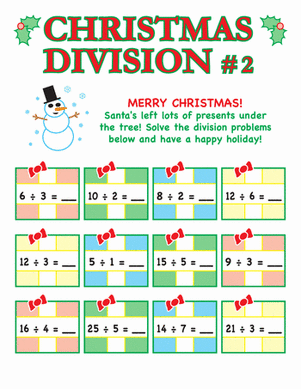Christmas Division #2
Worksheet
Christmas Division #2
Keep the learning going over the holiday break!Santa has brought some colorful presents filled with simple division problems for your child to solve.
Math
Worksheet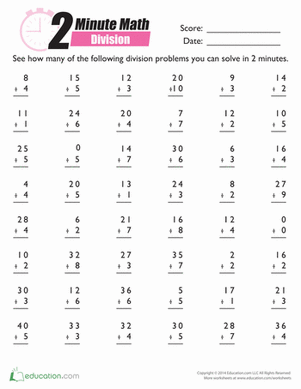2-Minute Math: Division
Worksheet
2-Minute Math: Division
Math minutes are a thrilling, fast-paced way to get lots of arithmetic practice. Race against the clock to solve these division problems in two minutes.
Worksheet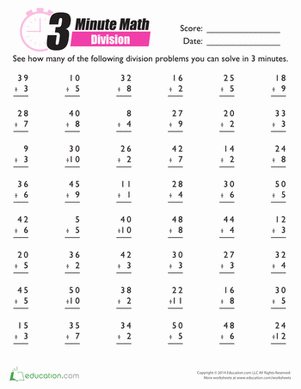3-Minute Division
Worksheet
3-Minute Division
On your mark, get set, go! Start an exciting math race with this worksheet filled with division problems that your little one must solve in under three minutes.
Worksheet

## Lesson PlansMusical Chair Multiplication
Lesson plan
Musical Chair Multiplication
Get your students moving while practicing their multiplication facts! Bring an old favorite to the classroom as students move about and work problems out. This is a fun way to incorporate movement and learning with a twist.
Math
Lesson plan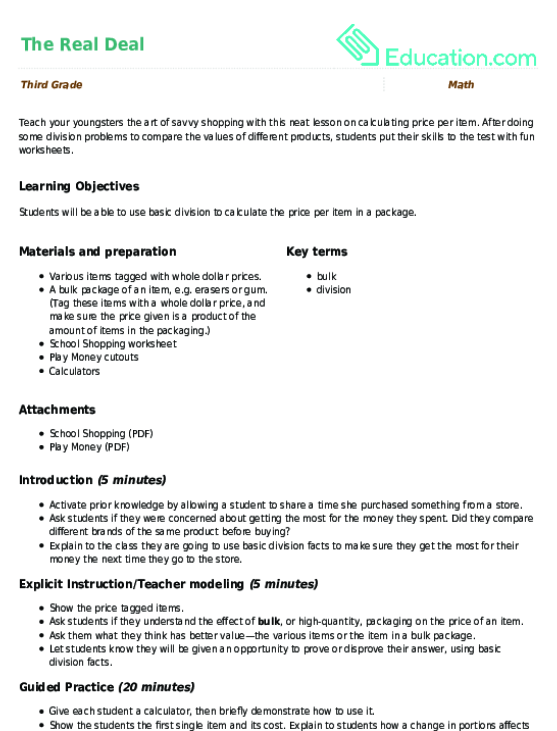The Real Deal
Lesson plan
The Real Deal
Teach your youngsters the art of savvy shopping with this neat lesson on calculating price per item. After doing some division problems to compare the values of different products, students put their skills to the test with fun worksheets.
Math
Lesson plan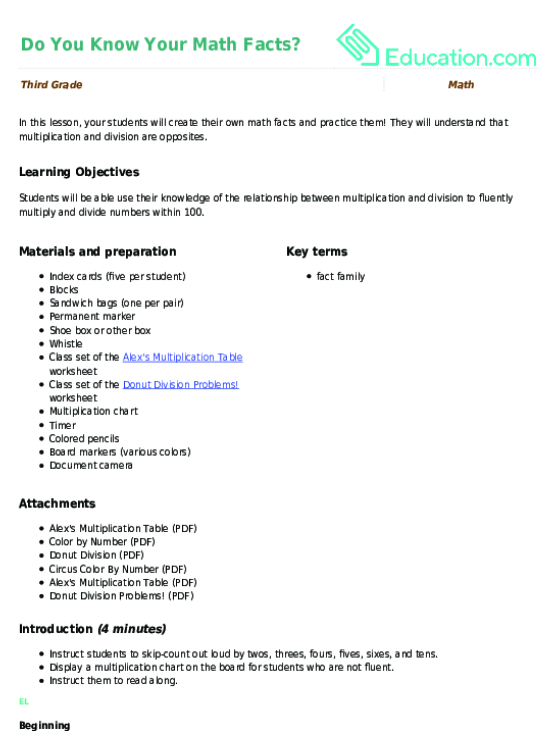Do You Know Your Math Facts?
Lesson plan
Do You Know Your Math Facts?
In this lesson, your students will create their own math facts and practice them! They will understand that multiplication and division are opposites.
Math
Lesson plan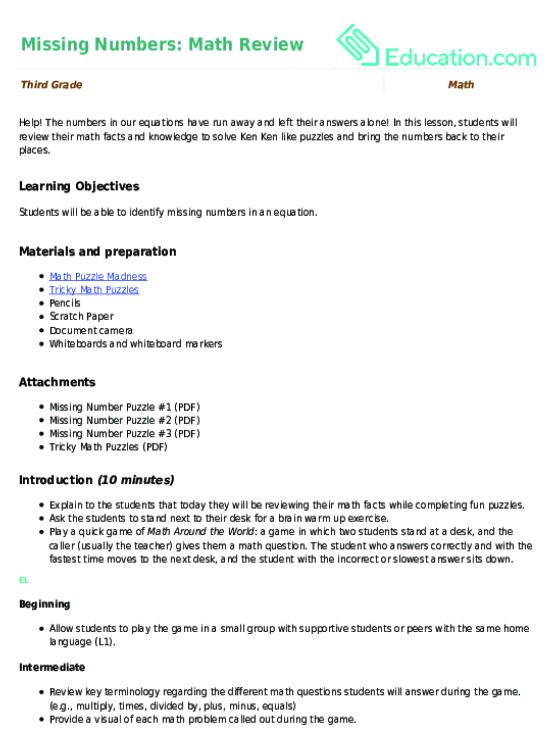Missing Numbers: Math Review
Lesson plan
Missing Numbers: Math Review
Help! The numbers in our equations have run away and left their answers alone! In this lesson, students will review their math facts and knowledge to solve Ken Ken like puzzles and bring the numbers back to their places.
Math
Lesson planHands-On Multiplication
Lesson plan
Hands-On Multiplication
Students get hands-on practice playing this math multiplication game with their hands!
Math
Lesson planMultiplication Cups Competition
Lesson plan
Multiplication Cups Competition
Motivate your students by engaging them in a competition! In this lesson, your students will review and practice multiplication strategies.
Math
Lesson plan

## Workbooks

No workbooks found for this common core node.

## Games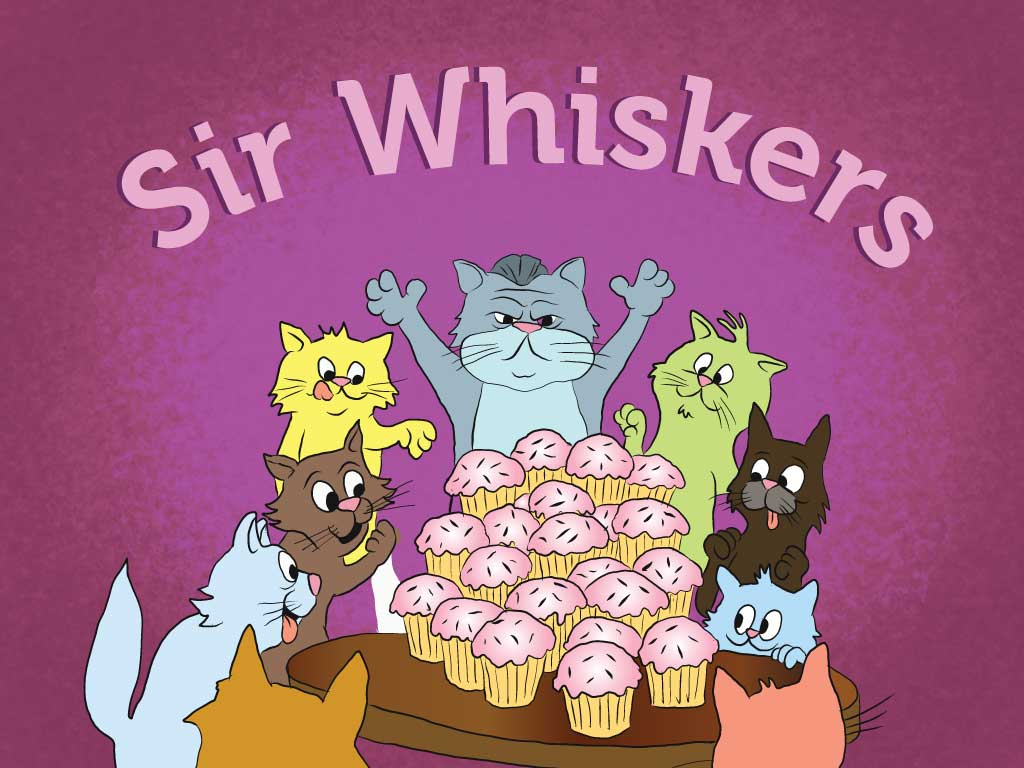Sir Whiskers Division Story
Story
Sir Whiskers Division Story
Kids learn all about division vocabulary and strategies with this silly story about a cat crew.
Math
Story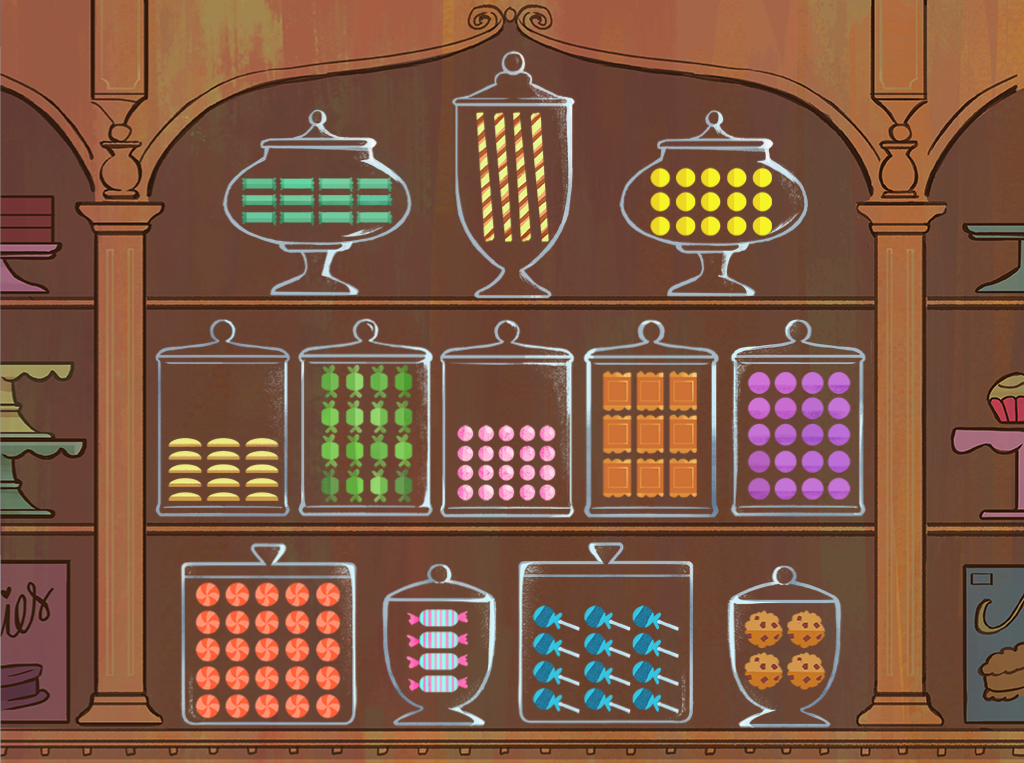Candy Shop Arrays
Game
Candy Shop Arrays
Sweet multiplication! Kids practice finding products using arrays in this delicious math game.
Math
Game

## ExercisesMultiply by Numbers 2-5
Exercise
Multiply by Numbers 2-5
Multiply by Numbers 2-5 will help students practice this key third grade skill. Try our free exercises to build knowledge and confidence.
Math
Exercise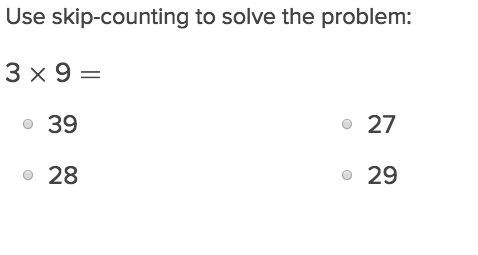One-Digit Multiplication with Skip-Counting
Exercise
One-Digit Multiplication with Skip-Counting
Students can incorporate skip counting as a tool used in verifying their correct multiplication answers.
Math
Exercise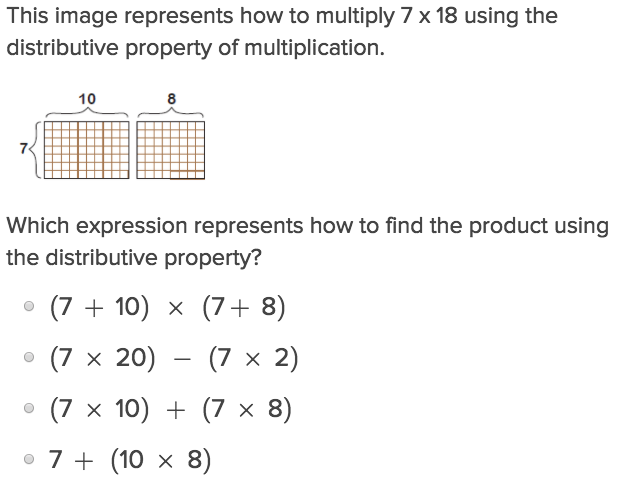Multiplication and the Distributive Property
Exercise
Multiplication and the Distributive Property
As students grow more comfortable with the order of operations, they’ll need this exercise to introduce them to working with one digit distributive properties.
Math
Exercise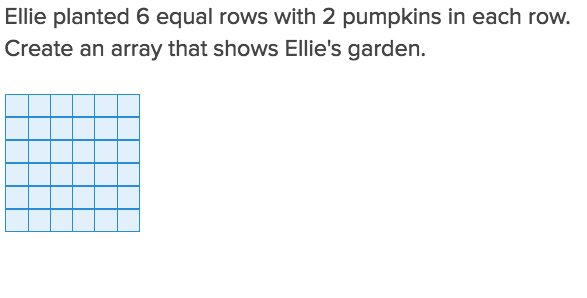Multiply by Numbers 6-9
Exercise
Multiply by Numbers 6-9
Multiply by Numbers 6-9 will help students practice this key third grade skill. Try our free exercises to build knowledge and confidence.
Math
Exercise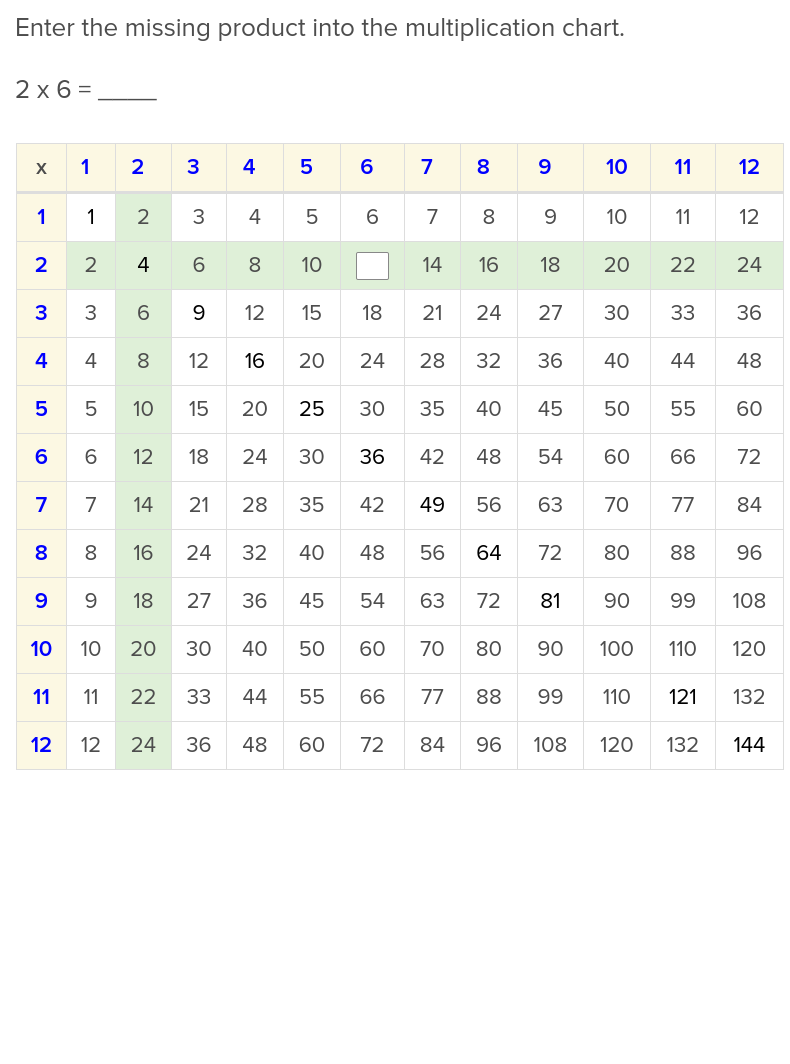Multiply by 2
Exercise
Multiply by 2
Students who are attracted to the difference between odds and evens will like studying these facts about multiplying 2s.
Math
Exercise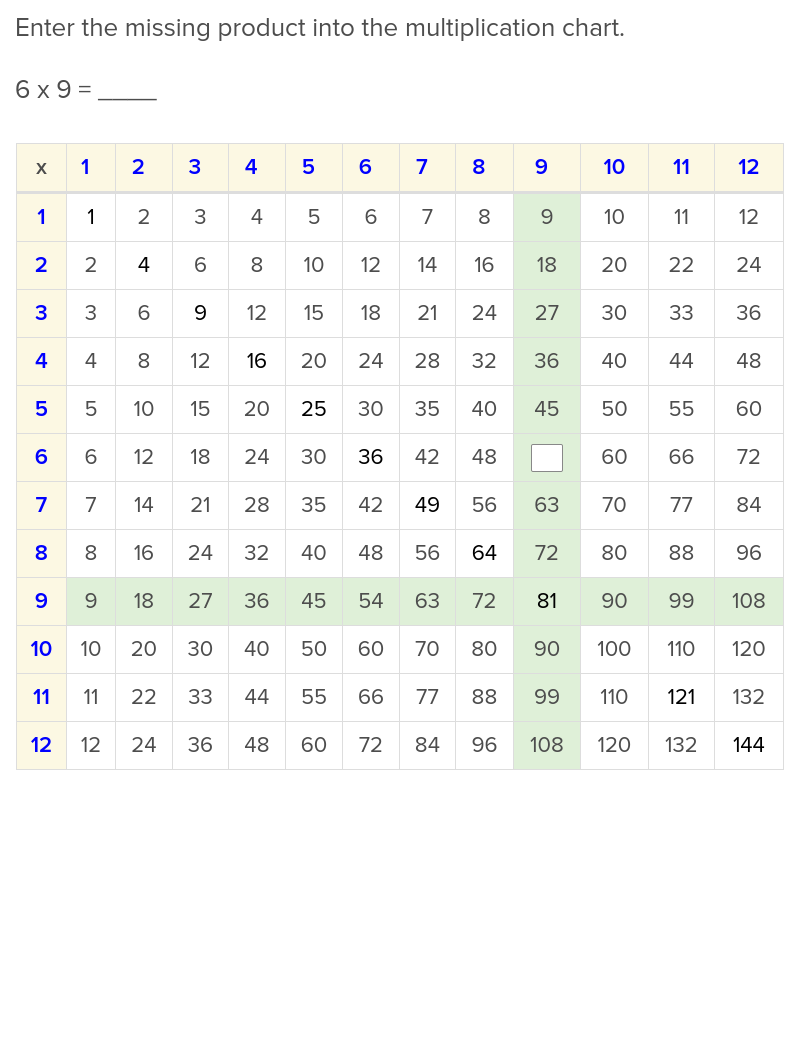Multiply by 9
Exercise
Multiply by 9
You can teach your students the hand trick in conjunction with these facts about multiplying 9s.